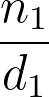# Equivalent Fractions Calculator

Created by Wojciech Sas, PhD candidate
Reviewed by Bogna Szyk and Jack Bowater
Last updated: Nov 29, 2021

This equivalent fractions calculator is a great tool that helps you find equivalent fractions for any fraction you wish. This is the right place to learn what is an equivalent fraction of x, or how to find equivalent fractions. Wondering whether two fractions are equivalent? You can get the answer to this question as well!

## What is an equivalent fraction?

A fraction is a ratio of two numbers, the numerator, A and the denominator, B. As you already know from the ratio calculator, the ratio is equal to some specific value, x, where x = A/B.

The equivalent fraction definition tells us that any two fractions, A/B and C/D, are equivalent if they are equal to the same value. We can find whether or not two fractions are equivalent by checking one of these conditions:

• A = C and B = D
• A * D = B * C
• C = k * A and D = k * B, for any number k

Converting two equivalent fractions to percentages will give us exactly the same number.

## How to find equivalent fractions?

For any fraction, there are infinitely many equivalent fractions. It's convenient to present such values as a ratio of two integer numbers. That's how we divide things in everyday life, e.g., cutting a pizza into pieces (and taking a few of them).

As an example, let's check how to find equivalent fractions of 4/6. We can follow these steps:

1. Make sure both numerator and denominator are integers. If not, start multiplying both numbers by 10 until there are no more decimal digits. In our case, both 4 and 6 are already integers.

2. Let's find the fraction in its simplest form. To do so, evaluate the greatest common factor of the numerator and the denominator. Here, the GCF of 4 and 6 is 2, so 4/6 is an equivalent fraction of 2/3, and the latter is the simplest form of this ratio. Therefore, 2/3 is our base.

3. Multiply the base by consecutive natural numbers:

• 2·2/2·3 = 4/6
• 3·2/3·3 = 6/9
• 4·2/4·3 = 8/12
• etc...
1. All of the values are equivalent fractions of 2/3, and are also equivalent to 4/6, the number we started with.

The procedure is straightforward, but what if you want to find 20 or 30 equivalent fractions? Well, it gets pretty time-consuming. However, if you use our equivalent fractions calculator, we can save you all of that hassle.

You can always convert any decimal to a fraction, and then work out what are its equivalent fractions from there.

## How to use equivalent fractions calculator?

There are two different modes you can choose from. The first one helps you find as many fractions that are equivalent to your number as you want. Check the previous section for how these calculations work.

The second option helps you find if two fractions are equivalent. For instance, is 13/16 an equivalent fraction to 3/4? Let's check one of the conditions, e.g. multiply the numerator and denominator from the opposing fractions:

• 13 * 4 = 52
• 16 * 3 = 48

The outcomes are different, so the fractions 13/16 and 3/4 are NOT equivalent. What about 12/16 and 3/4?

• 12 * 4 = 48
• 16 * 3 = 48

This time, both numbers are the same, so 12/16 IS equivalent to 3/4. By using this equivalent fraction calculator, you can also see how to obtain one fraction from another!

## What are equivalent fractions of…

Here is a list of a few fractions and their equivalent fractions:

• Equivalent fractions of 1/1: 2/2, 3/3, 4/4, 5/5

• Equivalent fractions of 1/2: 2/4, 3/6, 4/8, 5/10

• Equivalent fractions of 1/3: 2/6, 3/9, 4/12, 5/15

• Equivalent fractions of 2/3: 4/6, 6/9, 8/12, 10/15

• Equivalent fractions of 1/4: 2/8, 3/12, 4/16, 5/20

• Equivalent fractions of 3/4: 6/8, 9/12, 12/16, 15/20

• Equivalent fractions of 1/5: 2/10, 3/15, 4/20, 5/25

• Equivalent fractions of 2/5: 4/10, 6/15, 8/20, 10/25

• Equivalent fractions of 3/5: 6/10, 9/15, 12/20, 15/25

• Equivalent fractions of 4/5: 8/10, 12/15, 16/20, 20/25

• Equivalent fractions of 1/6: 2/12, 3/18, 4/24, 5/30

• Equivalent fractions of 5/6: 10/12, 15/18, 20/24, 25/30

Wojciech Sas, PhD candidateChoose what you want to see
Find equivalent fractions of...
a fraction in
simple fraction form
Numerator (n₁)
Denominator (d₁)
Number of equivalent fractions
People also viewed…

### Car heat

The hot car calculator shows you how fast the interior of a car heats up during a summers day.

### Multiplicative inverse

The multiplicative inverse calculator will take your decimal, simple fraction, or mixed number and find its multiplicative inverse, i.e., the number that gives 1 when multiplied by your input.

### Rectangular pyramid volume

Use the rectangular pyramid volume calculator to determine the volume and surface area of a rectangular pyramid.

### Sunbathing

Do you always remember to put on sunscreen before going outside? Are you sure that you use enough? The Sunbathing Calculator ☀ will tell you when's the time to go back under an umbrella not to suffer from a sunburn!